May 16, 2018

# Using Linear Regression for Predictive Modeling in R

In R programming, predictive models are extremely useful for forecasting future outcomes and estimating metrics that are impractical to measure. For example, data scientists could use predictive models to forecast crop yields based on rainfall and temperature, or to determine whether patients with certain traits are more likely to react badly to a new medication.

Before we talk about linear regression specifically, let’s remind ourselves what a typical data science workflow might look like. A lot of the time, we’ll start with a question we want to answer, and do something like the following:

1. Collect some data relevant to the problem (more is almost always better).
2. Clean, augment, and preprocess the data into a convenient form, if needed.
3. Conduct an exploratory analysis of the data to get a better sense of it.
4. Using what you find as a guide, construct a model of some aspect of the data.
5. Use the model to answer the question you started with, and validate your results.

Linear regression is one of the simplest and most common supervised machine learning algorithms that data scientists use for predictive modeling. In this post, we’ll use linear regression to build a model that predicts cherry tree volume from metrics that are much easier for folks who study trees to measure.

We’ll use R in this blog post to explore this data set and learn the basics of linear regression. If you’re new to learning the R language, we recommend our R Fundamentals and R Programming: Intermediate courses from our R Data Analyst path. It will also help to have some very basic statistics knowledge, but if you know what a mean and standard deviation are, you’ll be able to follow along. If you want to practice building the models and visualizations yourself, we’ll be using the following R packages:

• data sets This package contains a wide variety of practice data sets. We’ll be using one of them, “trees”, to learn about building linear regression models.
• ggplot2 We’ll use this popular data visualization package to build plots of our models.
• GGally This package extends the functionality of ggplot2. We’ll be using it to create a plot matrix as part of our initial exploratory data visualization.
• scatterplot3d We’ll use this package for visualizing more complex linear regression models with multiple predictors.

## How do they measure tree volume, anyway?

The trees data set is included in base R’s datasets package, and it’s going to help us answer this question. Since we’re working with an existing (clean) data set, steps 1 and 2 above are already done, so we can skip right to some preliminary exploratory analysis in step 3. What does this data set look like?

data(trees) ## access the data from R’s datasets package
head(trees) ## look at the first several rows of the data
Girth Height Volume
8.3 70 10.3
8.6 65 10.3
8.8 63 10.2
10.5 72 16.4
10.7 81 18.8
10.8 83 19.7
str(trees) ## look at the structure of the variables
 $Girth : num 8.3 8.6 8.8 10.5 10.7 10.8 11 11 11.1 11.2 …$ Height: num 70 65 63 72 81 83 66 75 80 75 … $Volume: num 10.3 10.3 10.2 16.4 18.8 19.7 15.6 18.2 22.6 19.9 … This data set consists of 31 observations of 3 numeric variables describing black cherry trees: • The trunk girth (in) • height (ft) • volume (ft3) These metrics are useful information for foresters and scientists who study the ecology of trees. It’s fairly simple to measure tree heigh and girth using basic forestry tools, but measuring tree volume is a lot harder. If you don’t want to actually cut down and dismantle the tree, you have to resort to some technically challenging and time-consuming activities like climbing the tree and making precise measurements. It would be useful to be able to accurately predict tree volume from height and/or girth.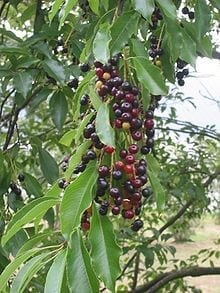To decide whether we can make a predictive model, the first step is to see if there appears to be a relationship between our predictor and response variables (in this case girth, height, and volume). Let’s do some exploratory data visualization. We’ll use the ggpairs() function from the GGally package to create a plot matrix to see how the variables relate to one another. ggpairs(data=trees, columns=1:3, title="trees data")The ggpairs() function gives us scatter plots for each variable combination, as well as density plots for each variable and the strength of correlations between variables. If you’ve used ggplot2 before, this notation may look familiar: GGally is an extension of ggplot2 that provides a simple interface for creating some otherwise complicated figures like this one. As we look at the plots, we can start getting a sense of the data and asking questions. The correlation coefficients provide information about how close the variables are to having a relationship; the closer the correlation coefficient is to 1, the stronger the relationship is. The scatter plots let us visualize the relationships between pairs of variables. Scatter plots where points have a clear visual pattern (as opposed to looking like a shapeless cloud) indicate a stronger relationship. Our questions: Which predictor variables seem related to the response variable? From looking at the ggpairs() output, girth definitely seems to be related to volume: the correlation coefficient is close to 1, and the points seem to have a linear pattern. There may be a relationship between height and volume, but it appears to be a weaker one: the correlation coefficient is smaller, and the points in the scatter plot are more dispersed. What is the shape of the relationship between the variables? The relationship appears to be linear; from the scatter plot, we can see that the tree volume increases consistently as the tree girth increases. Is the relationship strong, or is noise in the data swamping the signal? The relationship between height and volume isn’t as clear, but the relationship between girth and volume seems strong. Now that we have a decent overall grasp of the data, we can move on to step 4 and do some predictive modeling. ## Forming a hypothesis A hypothesis is an educated guess about what we think is going on with our data. In this case, let’s hypothesize that cherry tree girth and volume are related. Every hypothesis we form has an opposite: the “null hypothesis” (H0). Here, our null hypothesis is that girth and volume aren’t related. In statistics, the null hypothesis is the one we use our data to support or reject; we can’t ever say that we “prove” a hypothesis. We call the hypothesis that girth and volume are related our “alternative” hypothesis (Ha). To summarize: H0 : There is no relationship between girth and volume Ha: There is some relationship between girth and volume Our linear regression model is what we will use to test our hypothesis. If we find strong enough evidence to reject H0, we can then use the model to predict cherry tree volume from girth. ## Building blocks of a linear regression model Linear regression describes the relationship between a response variable (or dependent variable) of interest and one or more predictor (or independent) variables. It helps us to separate the signal (what we can learn about the response variable from the predictor variable) from the noise (what we can’t learn about the response variable from the predictor variable). We’ll dig deeper into how the model does this as we move along.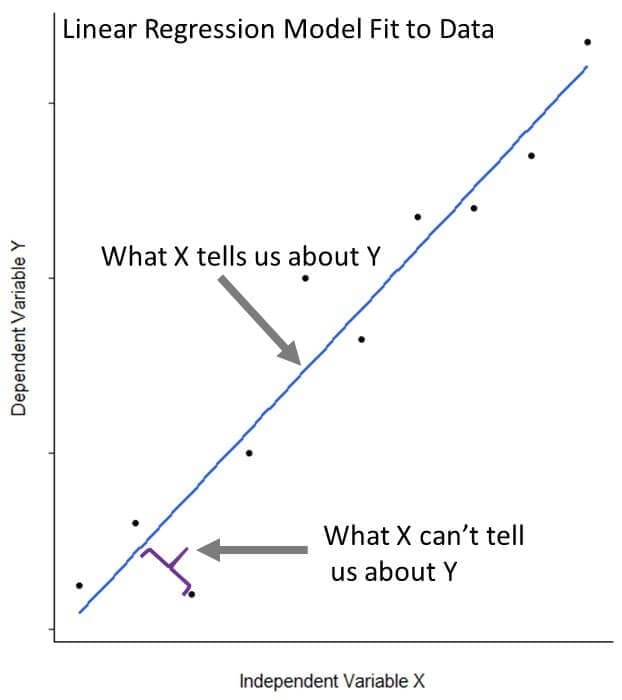Let’s dive right in and build a linear model relating tree volume to girth. R makes this straightforward with the base function lm(). fit_1 <- lm(Volume ~ Girth, data = trees) The lm() function fits a line to our data that is as close as possible to all 31 of our observations. More specifically, it fits the line in such a way that the sum of the squared difference between the points and the line is minimized; this method is known as “minimizing least squares.” Even when a linear regression model fits data very well, the fit isn’t perfect. The distances between our observations and their model-predicted value are called residuals.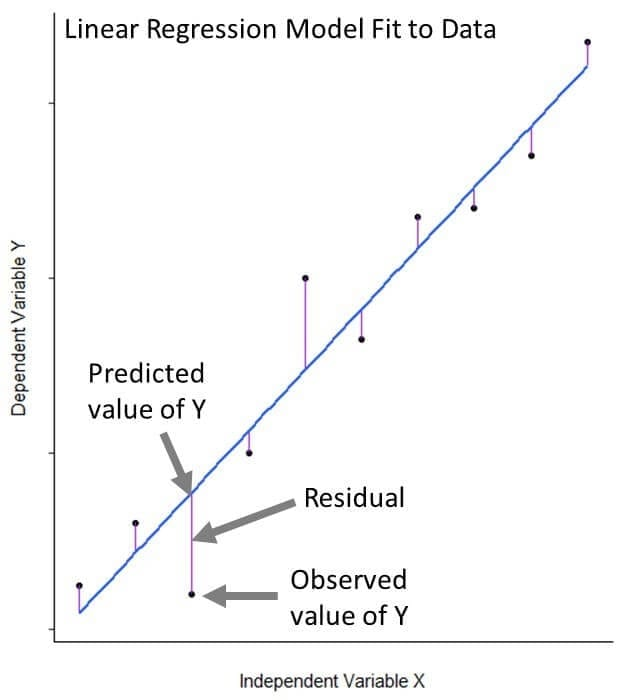Mathematically, can we write the equation for linear regression as: Y ≈ β0 + β1X + ε • The Y and X variables are the response and predictor variables from our data that we are relating to eachother • β0 is the model coefficient that represents the model intercept, or where it crosses the y axis • β1 is the model coefficient that represents the model slope, the number that gives information about the steepness of the line and its direction (positive or negative) • ε is the error term that encompasses variability we cannot capture in the model (what X cannot tell us about Y) In the case of our example: Tree Volume ≈ Intercept + Slope(Tree Girth) + Error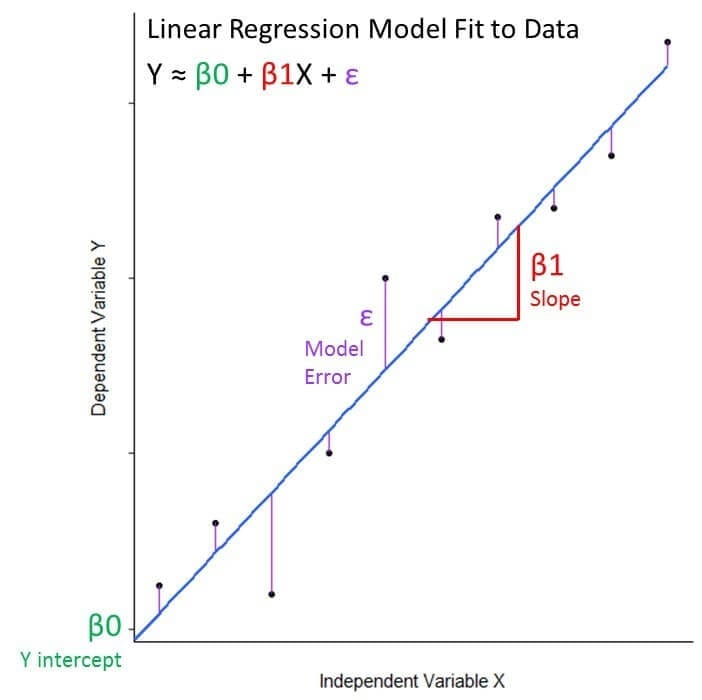The lm() function estimates the intercept and slope coefficients for the linear model that it has fit to our data. With a model in hand, we can move on to step 5, bearing in mind that we still have some work to do to validate the idea that this model is actually an appropriate fit for the data. ## Can we use this model to make predictions? Whether we can use our model to make predictions will depend on: 1. Whether we can reject the null hypothesis that there is no relationship between our variables. 2. Whether the model is a good fit for our data. Let’s call the output of our model using summary(). The model output will provide us with the information we need to test our hypothesis and assess how well the model fits our data. summary(fit_1)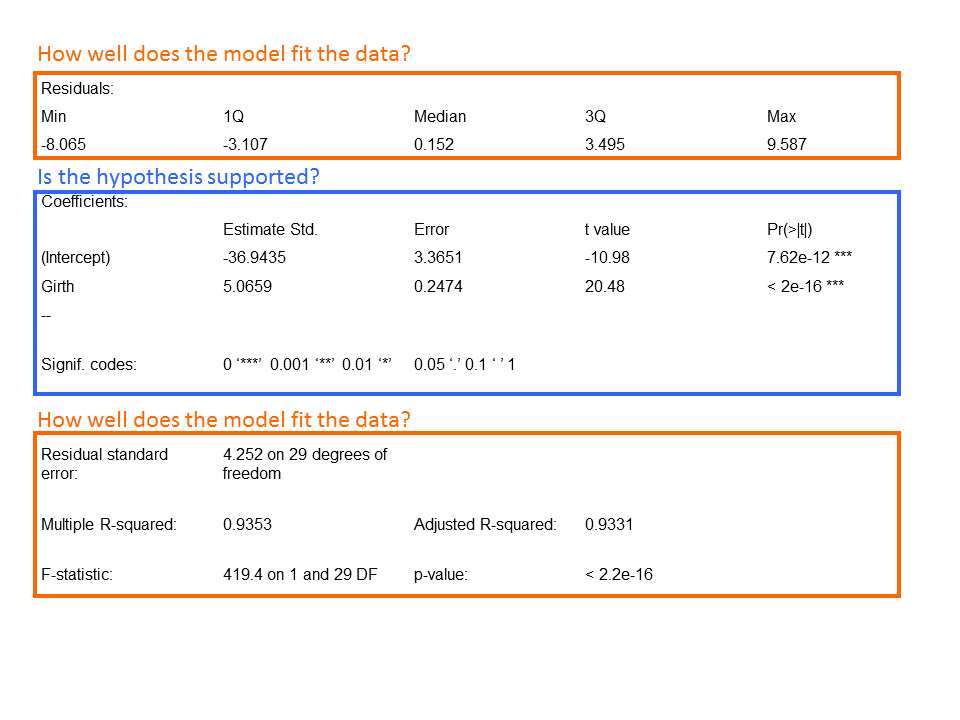Let’s walk through the output to answer each of these questions. ### Is the hypothesis supported? Coefficients: Estimate and Std. Error: • The intercept in our example is the expected tree volume if the value of girth was zero. Of course we cannot have a tree with negative volume, but more on that later. • The slope in our example is the effect of tree girth on tree volume. We see that for each additional inch of girth, the tree volume increases by 5.0659 ft3. • The coefficient standard errors tell us the average variation of the estimated coefficients from the actual average of our response variable. t value: Pr(>|t|): • This number is the p-value, defined as the probability of observing any value equal or larger than t if H0 is true. The larger the t statistic, the smaller the p-value. Generally, we use 0.05 as the cutoff for significance; when p-values are smaller than 0.05, we reject H0. We can reject the null hypothesis in favor of believing there to be a relationship between tree width and volume. ### How well does the model fit the data? Residuals: • This section of the output provides us with a summary of the residuals (recall that these are the distances between our observation and the model), which tells us something about how well our model fit our data. The residuals should have a pretty symmetrical distribution around zero. Generally, we’re looking for the residuals to be normally distributed around zero (i.e. a bell curve distribution), but the important thing is that there’s no visually obvious pattern to them, which would indicate that a linear model is not appropriate for the data. We can make a histogram to visualize this using ggplot2. ggplot(data=trees, aes(fit_1$residuals)) +
geom_histogram(binwidth = 1, color = "black", fill = "purple4") +
theme(panel.background = element_rect(fill = "white"),
axis.line.x=element_line(),
axis.line.y=element_line()) +
ggtitle("Histogram for Model Residuals")Our residuals look pretty symmetrical around 0, suggesting that our model fits the data well. Residual standard error:

• This term represents the average amount that our response variable measurements deviate from the fitted linear model (the model error term).

Degrees of freedom (DoF):

• Discussion of degrees of freedom can become rather technical. For the purposes of this post, it is sufficient to think of them as the number of independent pieces of information that were used to calculate an estimate. DoF are related to, but not the same as, the number of measurements.

Multiple R-squared:

• The R2 value is a measure of how close our data are to the linear regression model. R2 values are always between 0 and 1; numbers closer to 1 represent well-fitting models. R2 always increases as more variables are included in the model, and so adjusted R2 is included to account for the number of independent variables used to make the model.

F statistic:

• This test statistic tells us if there is a relationship between the dependent and independent variables we are testing. Generally, a large F indicates a stronger relationship.

p-value:

• This p-value is associated with the F statistic, and is used to interpret the significance for the whole model fit to our data.

Let’s have a look at our model fitted to our data for width and volume. We can do this by using ggplot() to fit a linear model to a scatter plot of our data:

ggplot(data = trees, aes(x = Girth, y = Volume)) +
geom_point() +
stat_smooth(method = "lm", col = "dodgerblue3") +
theme(panel.background = element_rect(fill = "white"),
axis.line.x=element_line(),
axis.line.y=element_line()) +
ggtitle("Linear Model Fitted to Data")The gray shading around the line represents a confidence interval of 0.95, the default for the stat_smooth() function, which smoothes data to make patterns easier to visualize. This 0.95 confidence interval is the probability that the true linear model for the girth and volume of all black cherry trees will lie within the confidence interval of the regression model fitted to our data. Even though this model fits our data quite well, there is still variability within our observations.

This is because the world is generally untidy. In our model, tree volume is not just a function of tree girth, but also of things we don’t necessarily have data to quantify (individual differences between tree trunk shape, small differences in foresters’ trunk girth measurement techniques). Sometimes, this variability obscures any relationship that may exist between response and predictor variables. But here, the signal in our data is strong enough to let us develop a useful model for making predictions.

## Using our simple linear model to make predictions

Our model is suitable for making predictions! Tree scientists everywhere rejoice. Let’s say we have girth, height and volume data for a tree that was left out of the data set. We can use this tree to test our model.

Girth Height Volume
18.2 in 72 ft 46.2 ft3

How well will our model do at predicting that tree’s volume from its girth? We’ll use the predict() function, a generic R function for making predictions from modults of model-fitting functions. predict() takes as arguments our linear regression model and the values of the predictor variable that we want response variable values for.

predict(fit_1, data.frame(Girth = 18.2))

Our volume prediction is 55.2 ft3. This is close to our actual value, but it’s possible that adding height, our other predictive variable, to our model may allow us to make better predictions.

## Adding more predictors: multiple linear regression

Maybe we can improve our model’s predictive ability if we use all the information we have available (width and height) to make predictions about tree volume. It’s important that the five-step process from the beginning of the post is really an iterative process – in the real world, you’d get some data, build a model, tweak the model as needed to improve it, then maybe add more data and build a new model, and so on, until you’re happy with the results and/or confident that you can’t do any better. We could build two separate regression models and evaluate them, but there are a few problems with this approach. First, imagine how cumbersome it would be if we had 5, 10, or even 50 predictor variables. Second, two predictive models would give us two separate predictions for volume rather than the single prediction we’re after. Perhaps most importantly, building two separate models doesn’t let us account for relationships among predictors when estimating model coefficients. In our data set, we suspect that tree height and girth are correlated based on our initial data exploration. As we’ll begin to see more clearly further along in this post, ignoring this correlation between predictor variables can lead to misleading conclusions about their relationships with tree volume. A better solution is to build a linear model that includes multiple predictor variables. We can do this by adding a slope coefficient for each additional independent variable of interest to our model.

Tree Volume ≈ Intercept + Slope1(Tree Girth) + Slope2(Tree Height) + Error

This is easy to do with the lm() function: We just need to add the other predictor variable.

fit_2 <- lm(Volume ~ Girth + Height, data = trees)
summary(fit_2)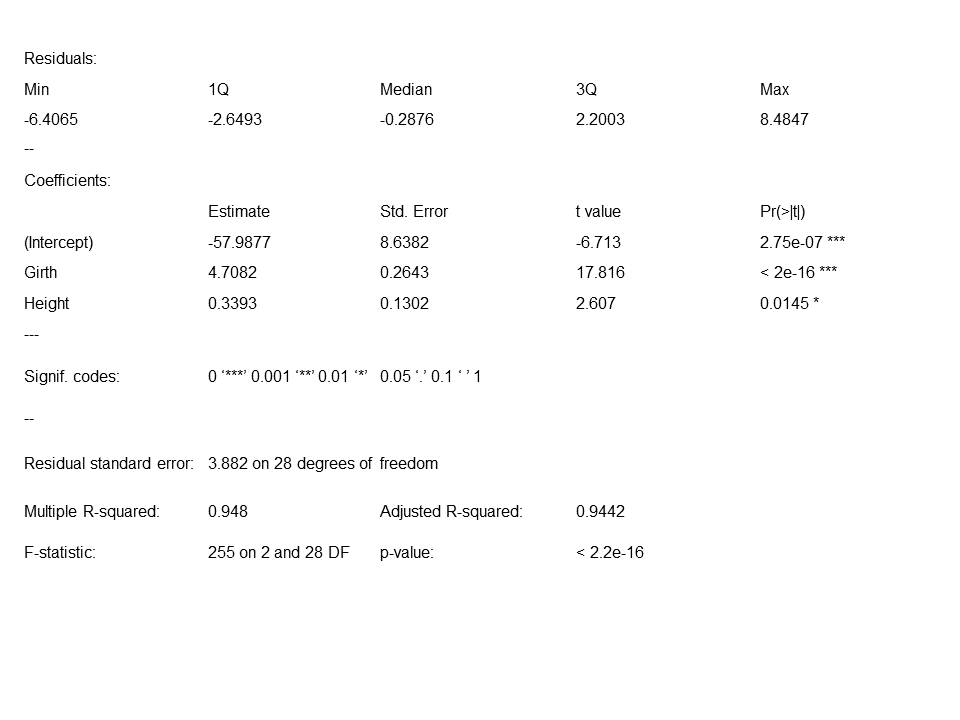We can see from the model output that both girth and height are significantly related to volume, and that the model fits our data well. Our adjusted R2 value is also a little higher than our adjusted R2 for model fit_1. Since we have two predictor variables in this model, we need a third dimension to visualize it. We can create a nice 3d scatter plot using the package scatterplot3d: First, we make a grid of values for our predictor variables (within the range of our data). The expand.grid() function creates a data frame from all combinations of the factor variables.

Girth <- seq(9,21, by=0.5) ## make a girth vector
Height <- seq(60,90, by=0.5) ## make a height vector
pred_grid <- expand.grid(Girth = Girth, Height = Height)
## make a grid using the vectors

Next, we make predictions for volume based on the predictor variable grid:

pred_grid$Volume2 <-predict(fit_2, new = pred_grid) Now we can make a 3d scatterplot from the predictor grid and the predicted volumes: fit_2_sp <- scatterplot3d(pred_grid$Girth, pred_grid$Height, pred_grid$Volume2, angle = 60, color = "dodgerblue", pch = 1, ylab = "Hight (ft)", xlab = "Girth (in)", zlab = "Volume (ft3)" )

And finally overlay our actual observations to see how well they fit:

fit_2_sp$points3d(trees$Girth, trees$Height, trees$Volume, pch=16)Let’s see how this model does at predicting the volume of our tree. This time, we include the tree’s height since our model uses Height as a predictive variable:

predict(fit_2, data.frame(Girth = 18.2, Height = 72))

This time, we get a predicted volume of 52.13 ft3. This prediction is closer to our true tree volume than the one we got using our simple model with only girth as a predictor, but, as we’re about to see, we may be able to improve.

## Accounting for interactions

While we’ve made improvements, the model we just built still doesn’t tell the whole story. It assumes that the effect of tree girth on volume is independent from the effect of tree height on volume. This is clearly not the case, since tree height and girth are related; taller trees tend to be wider, and our exploratory data visualization indicated as much. Put another way, the slope for girth should increase as the slope for height increases. To account for this non-independence of predictor variables in our model, we can specify an interaction term, which is calculated as the product of the predictor variables.

Tree Volume ≈ Intercept + Slope1(Tree Girth) + Slope2(Tree Height) + Slope3(Tree Girth x Tree Height)+ Error

Once again, it’s easy to build this model using lm():

fit_3 <- lm(Volume ~ Girth * Height, data = trees)
summary(fit_3)

Note that the “Girth * Height” term is shorthand for “Girth + Height + Girth * Height” in our model.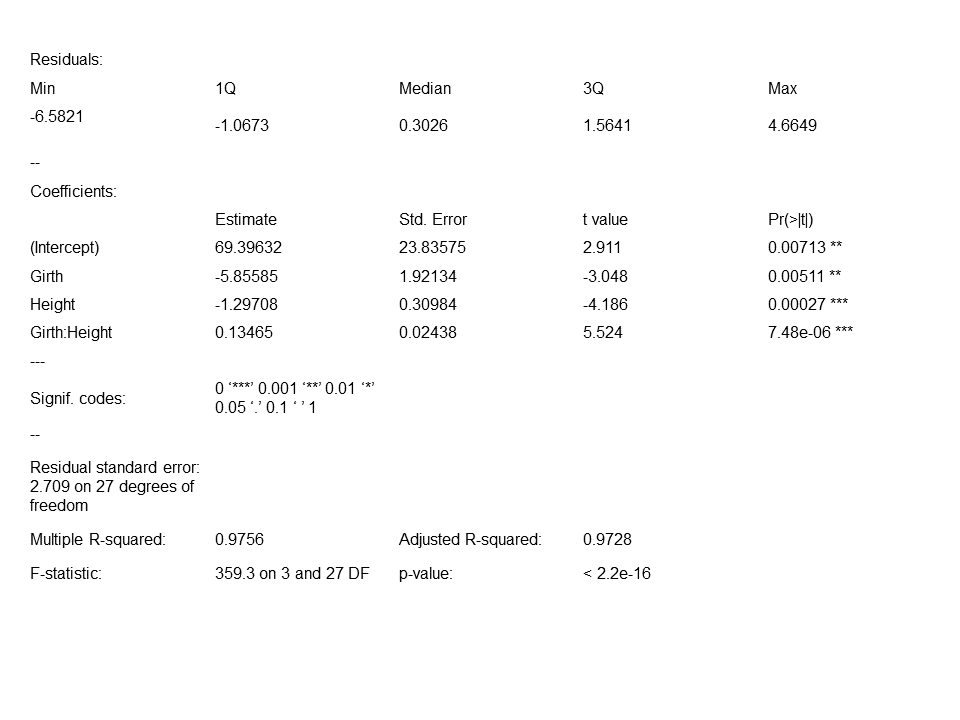As we suspected, the interaction of girth and height is significant, suggesting that we should include the interaction term in the model we use to predict tree volume. This decision is also supported by the adjusted R2 value close to 1, the large value of F and the small value of p that suggest our model is a very good fit for the data. Let’s have a look at a scatter plot to visualize the predicted values for tree volume using this model. We can use the same grid of predictor values we generated for the fit_2 visualization:

Girth <- seq(9,21, by=0.5)
Height <- seq(60,90, by=0.5)
pred_grid <- expand.grid(Girth = Girth, Height = Height)

Similarly to how we visualized the fit_2 model, we will use the fit_3 model with the interaction term to predict values for volume from the grid of predictor variables:

pred_grid$Volume3 <-predict(fit_3, new = pred_grid) Now we make a scatter plot of the predictor grid and the predicted volumes: fit_3_sp <- scatterplot3d(pred_grid$Girth, pred_grid$Height, pred_grid$Volume3, angle = 60, color = "dodgerblue", pch = 1, ylab = "Hight (ft)", xlab = "Girth (in)", zlab = "Volume (ft3)")

Finally, we overlay our observed data:

fit_3_sp$points3d(trees$Girth, trees$Height, trees$Volume, pch=16)It’s a little hard to see in this picture, but this time our predictions lie on some curved surface instead of a flat plane. Now for the moment of truth: let’s use this model to predict our tree’s volume.

predict(fit_3, data.frame(Girth = 18.2, Height = 72))

Our predicted value using this third model is 45.89, the closest yet to our true value of 46.2 ft3.

## Some cautionary notes about predictive models

#### Keep the range of your data in mind

When using a model to make predictions, it’s a good idea to avoid trying to extrapolate to far beyond the range of values used to build the model. To illustrate this point, let’s try to estimate the volume of a small sapling (a young tree):

predict(fit_3, data.frame(Girth = 0.25, Height = 4))

We get a predicted volume of 62.88 ft3, more massive than the tall trees in our data set. Of course this doesn’t make sense. Keep in mind that our ability to make accurate predictions is constrained by the range of the data we use to build our models.

#### Avoid making a model that’s too specific to your data set

In the simple example data set we investigated in this post, adding a second variable to our model seemed to improve our predictive ability. However, when trying a variety of multiple linear regression models with many difference variables, choosing the best model becomes more challenging. If too many terms that don’t improve the model’s predictive ability are added, we risk “overfitting” our model to our particular data set.

A model that is overfit to a particular data set loses functionality for predicting future events or fitting different data sets and therefore isn’t terribly useful. While methods we used for assessing model validity in this post (adjusted R2, residual distributions) are useful for understanding how well your model fits your data, applying your model to different subsets of your data set can provide information about how well your model will perform in practice.

This method, known as “cross-validation”, is commonly used to test predictive models. In our example, we used each of our three models to predict the volume of a single tree. If we were building more complex models, however, we would want to withold a subset of the data for cross-validation.

## Next Steps

We used linear regression to build models for predicting continuous response variables from two continuous predictor variables, but linear regression is a useful predictive modeling tool for many other common scenarios.

As a next step, try building linear regression models to predict response variables from more than two predictor variables. Think about how you may decide which variables to include in a regression model; how can you tell which are important predictors? How might the relationships among predictor variables interfere with this decision?

Data sets in R that are useful for working on multiple linear regression problems include: airquality, iris, and mtcars. Another important concept in building models from data is augmenting your data with new predictors computed from the existing ones. This is called feature engineering, and it’s where you get to use your own expert knowledge about what else might be relevant to the problem.

For example, if you were looking at a database of bank transactions with timestamps as one of the variables, it’s possible that day of the week might be relevant to the question you wanted to answer, so you could compute that from the timestamp and add it to the database as a new variable. This is a complicated topic, and adding more predictor variables isn’t always a good idea, but it’s something you should keep in mind as you learn more about modeling. In the trees data set used in this post, can you think of any additional quantities you could compute from girth and height that would help you predict volume? (Hint: think back to when you learned the formula for the volumes of various geometric shapes, and think about what a tree looks like.)

Finally, although we focused on continuous data, linear regression can be extended to make predictions from categorical variables, too. Try using linear regression models to predict response variables from categorical as well as continuous predictor variables. There are a few data sets in R that lend themselves especially well to this exercise: ToothGrowth, PlantGrowth, and npk.

Our Data Analyst in R path covers all the skills you need to land a job, including:

• Data visualization with ggplot2
• Advanced data cleaning skills with tidyverse packages
• Important SQL skills for R users
• Fundamentals in statistics and probability
• ...and much more

There's nothing to install, no prerequisites, and no schedule.